Electrostatic Force – Coulomb’s Law

Electrostatic Force

All bodies are made of atoms. Atoms are composed of electrons, protons and neutrons. Electrons, protons and neutrons have mass but only the electron and proton have charge. The proton is positively charged and the electron is negatively charged.

If we place two electrons (negatively charged both) at a “r” distance, they repel each other with a “F” force. This force depends on the “r” distance between electrons and the charge of both. This “F” force is called: the Electrostatic Force.

If instead of electrons we use protons, there is also a force of repulsion, because the charges are equal. (both positive). The force will change to attractive if, instead of using two elements equally charged, we use two elements with different charges. (An electron and a proton).

The Electrostatic force will be a force of attraction or a force of repulsion depending on the type of charges:

– Negative charge (e1) with negative charge (e2) repels.
– Positive charge (P1) with positive charge (P2) repels.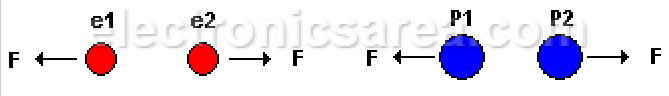– Positive (P1) charge with negative charge (e2) attract each other.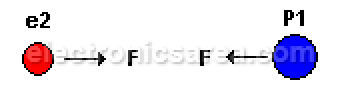– An electron (e) with a neutron (N) does not generate any force.
– A proton (P) with a neutron (N) does not generate any force.Remember that the neutron (N) is “neutral”, it has no charge. In conclusion:

Same charges repels each other,
different charges attract each other

Note: Protons and electrons have equal but oposite charges, however the mass of the proton is nearly 2000 times the mass of the electron.

Coulomb’s Law

The electrostatic force depends on the opposing electric charges and the distance that separates them. The value of this electrostatic force is given by Coulomb’s Law.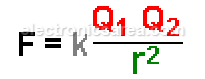where:

• F = electrostatic force that acts on each charge Q1 and Q2.
• K = a constant which depends on the unit system and the environment in which the charges are.
• r = distance between charges.

In the MKS system and in the vacuum: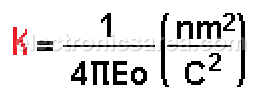where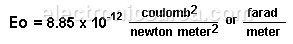In the MKS System: k = 9 x 109 newton-meter2/coulomb2

With this value of k, the charges will be expressed in coulombs and the distance (R) in meters, in order to obtain a resultant force in Newtons.

• If the charges are of opposite signs (+ and -), F will be negative, which indicates attraction.
• If the charges are of the same (+ and + or – and -), F will be positive, which indicates repulsion.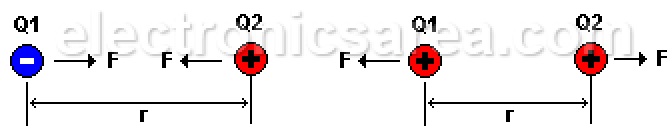Note: MKS System units: (Meters, Kilograms, Seconds).

electrostatic-force
coulombs-law

•
•
•
•
•
•
•
•
•
•
•
•
•
•
•
•
•
•